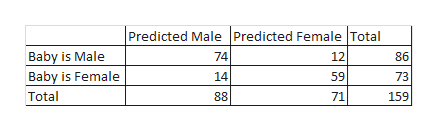# a. From a standard shuffled deck of cards, find the following probabilities:A draw of two cards without replacement that result in a face card then a fourb. The table below represents the results of gender prediction from an ultrasound Predicted MalePredicted FemaleBaby is Male7412Baby is Female1459Find the following probabilities:Correct PredictionPrediction is male given that the baby is male

Question
24 views

a. From a standard shuffled deck of cards, find the following probabilities:

1. A draw of two cards without replacement that result in a face card then a four

b. The table below represents the results of gender prediction from an ultrasound

 Predicted Male Predicted Female Baby is Male 74 12 Baby is Female 14 59

Find the following probabilities:

1. Correct Prediction
2. Prediction is male given that the baby is male
check_circle

Step 1

Solution:

a. From a standard shuffled deck of cards, find the following probabilities:

1. A draw of two cards without replacement that result in a face card then a four

Answer: Since there are 12 face cards in deck of cards and 4 cards four.

Therefore, the result is a face card and then a four is:

Step 2

b. Find the following probabilities:

a. Correct prediction.

We are given the following table:help_outlineImage TranscriptionclosePredicted Male Predicted Female Total Baby is Male 74 12 86 Baby is Female 14 59 73 Total 88 71 159 fullscreen
Step 3

Therefore, the probability of the corr...

### Want to see the full answer?

See Solution

#### Want to see this answer and more?

Solutions are written by subject experts who are available 24/7. Questions are typically answered within 1 hour.*

See Solution
*Response times may vary by subject and question.
Tagged in

### Other